New Delhi, India

# Mathematics Behind Linear Regression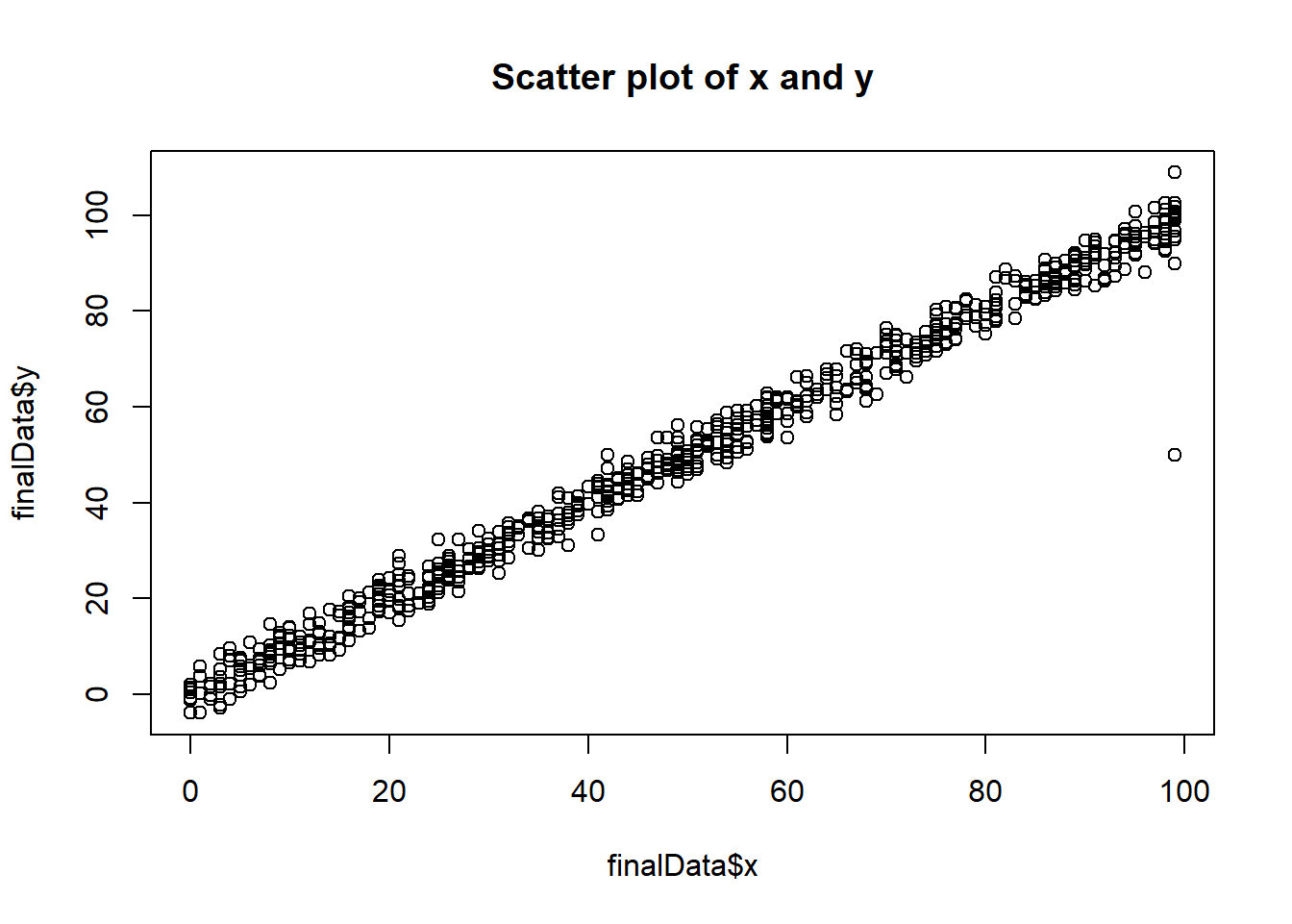# Mathematics Behind Linear Regression

Hi ML Enthusiasts! Today, we are going to learn about the mathematics behind linear regression.

## Basics of Linear Regression

The equation or curve that is used to represent the linear law is given as below:where i ranges from 1 to number of observations, N,

yi is the output variable, also called as predicted variable in Machine Learning terms,

xi is the input or explanatory variable,

m is defined as the slope or differential change in the value of y due to differential change in x,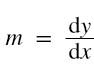and in case of multiple input variables or features,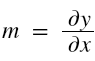and, c is called as intercept in mathematical terms. It can also be described as the variation in predicted value which can’t be explained by x or the input variable(s).

The above equation predicts value of y for each value of x.

## Mathematics behind linear regression – Practical approach

While making machine learning models, we divide the dataset into two parts: one part is called as the training data and the other as test data. We make the model learn using training dataset and then make predictions for the observations of test dataset. We then compare the predicted values with the real output values for the corresponding observations. For convenience, we will be usingto represent the actual values of the output variable andto represent the predicted values of the output.

### The Concept of Line of Best Fit

Line of Best Fit is the line which is made in such a way that the sum of square of errors (difference between the values predicted by it and the actual values of the output) is minimized. Thus, we can come out with many equations for making our predictive model. But, the model that we will choose has to be the model which corresponds to the line of best fit.

Now, the question arises how to find the line of Best Fit?

To answer this question, first of all understand the concept of Sum of Squares of Errors (SSE) which is given by the equation: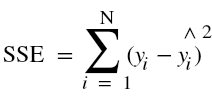We define the error as the difference between the actual value of y and the predicted value of y. This error is calculated for every observation and thus can be positive or negative. Thus, squaring of each error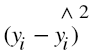is done to overcome the problem of cancellation of positive values with negative values of errors upon addition.

Coming back to our previous question, we follow the method of least squares to find the line of best fit.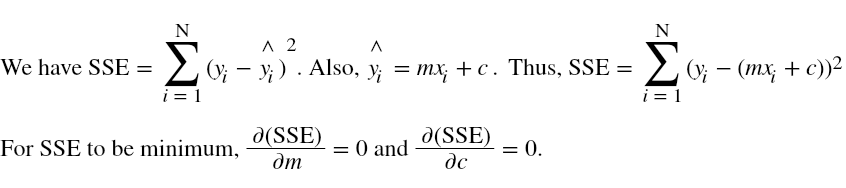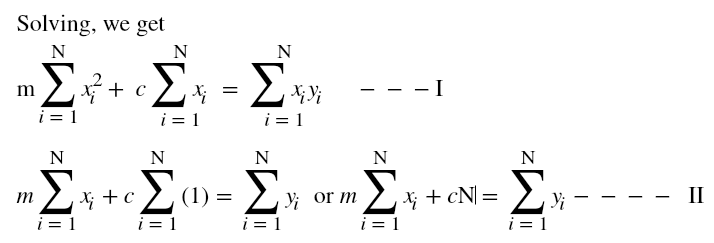Now, we have two equations and two unknowns, m and c. Solving the above two equations will result in those values of m and c which will give us an optimal solution and hence the equation for line of best fit.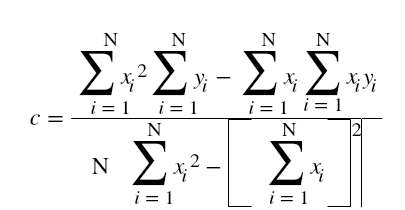or## Moore’s law and Linear Regression

Sometimes we encounter some problems that do not seem to be linear at first. The relation between them can be exponential and thus non-linear. In such a case, it is required to convert those non-linear problems into linear problems and then find out the ‘Line of best Fit”.

Let’s take the example of a transistor. Transistor count on integrated circuits doubles every 2 years. Thus, the relationship between y and x comes out to be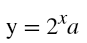where a is the default value or a constant. To make this equation linear, all we need to do is to take log on both the sides. The equation thus formed iswhich is linear.

## The Concept of R Squared

R Squared tells us about how much variation inis explained by xi in our model. R squared generally varies between 0 to 1 with the value of R squared equal to 1 signifying that our model is very accurate and 0 meaning model is very bad.Thus, if R squared = 1, we have SSE = 0 or a perfect model. If R squared = 0, we have SSE = SST or= mean of. Thus, the model comes out to be very bad. If it comes out to be a negative value, then we can say that the predicted value is worse than model having R squared = 0.

With this I conclude this tutorial. Stay tuned for more informative tutorials.

## 2 thoughts on “Mathematics Behind Linear Regression”

This site uses Akismet to reduce spam. Learn how your comment data is processed.